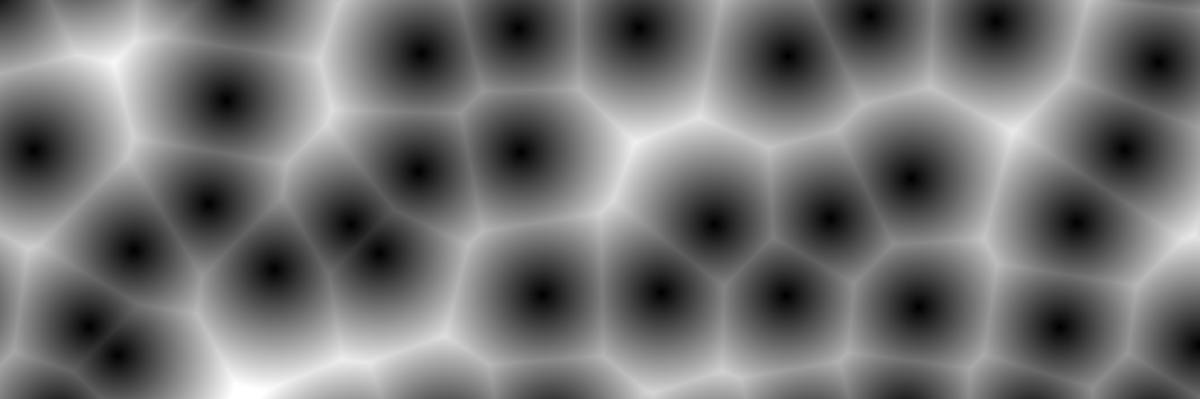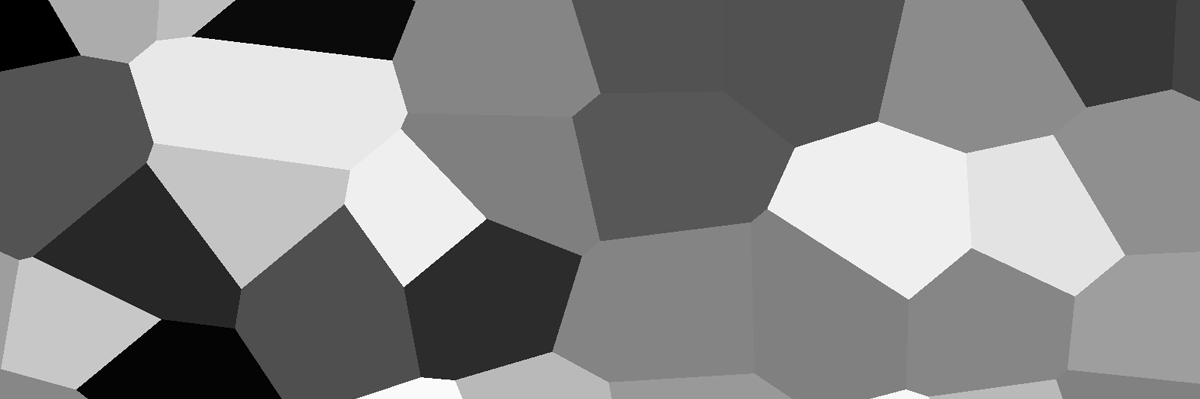# Voronoi and Worley (cellular) noise## Worley noise/cellular noise

Worley noise, or cellular noise, is a distance value pattern where every pixel gets a value based on the distance to the closest point from a defined set. This creates a pattern like the one above.

``````vec2 random(vec2 uv) {
return vec2(fract(sin(dot(uv.xy,
vec2(12.9898,78.233))) * 43758.5453123));
}

float worley(vec2 uv, float columns, float rows) {

vec2 index_uv = floor(vec2(uv.x * columns, uv.y * rows));
vec2 fract_uv = fract(vec2(uv.x * columns, uv.y * rows));

float minimum_dist = 1.0;

for (int y= -1; y <= 1; y++) {
for (int x= -1; x <= 1; x++) {
vec2 neighbor = vec2(float(x),float(y));
vec2 point = random(index_uv + neighbor);

vec2 diff = neighbor + point - fract_uv;
float dist = length(diff);
minimum_dist = min(minimum_dist, dist);
}
}

return minimum_dist;
}

void fragment() {
float worley = worley(UV, 3.0, 5.0);
COLOR = vec4(vec3(worley), 1.0);
}
``````

If you want the pattern to move you can add this to the loop. You will have to pass `TIME` from the fragment function. Play around with the different values for different results.

``````...
vec2 point = random(index_uv + neighbor);
float speed = 4.5;
point = vec2( cos(time * point.x * speed), sin(time * point.y * speed) ) * 0.5 + 0.5;
...
``````## Voronoi diagram

With the Worley noise as a base, we can make a Voronoi diagram. Instead of the fading distance value, each “cell” gets its own defined value.

``````vec2 voronoi(vec2 uv, float columns, float rows) {

vec2 index_uv = floor(vec2(uv.x * columns, uv.y * rows));
vec2 fract_uv = fract(vec2(uv.x * columns, uv.y * rows));

float minimum_dist = 1.0;
vec2 minimum_point;

for (int y= -1; y <= 1; y++) {
for (int x= -1; x <= 1; x++) {
vec2 neighbor = vec2(float(x),float(y));
vec2 point = random(index_uv + neighbor);

vec2 diff = neighbor + point - fract_uv;
float dist = length(diff);

if(dist < minimum_dist) {
minimum_dist = dist;
minimum_point = point;
}
}
}
return minimum_point;
}
void fragment() {
vec3 voronoi = vec3(voronoi(UV, 10.0, 5.0).r);
COLOR = vec4(voronoi, 1.0);
}
``````

This code is converted from The Book of Shaders article on Cellular Noise, where you can read more about how Voronoi diagrams and Worley noise (cellular noise) works.